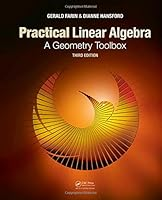# Practical Linear Algebra: A Geometry Toolbox, 3rd Edition## Book Description

Through many examples and real-world applications, Practical Linear Algebra: A Geometry Toolbox, Third Edition teaches undergraduate-level linear algebra in a comprehensive, geometric, and algorithmic way. Designed for a one-semester linear algebra course at the undergraduate level, the book gives instructors the option of tailoring the course for the primary interests: math, engineering, science, , and geometric .

New to the Third Edition

• More exercises and applications
• Coverage of singular value decomposition and its application to the pseudoinverse, principal components analysis, and image compression
• More attention to eigen-analysis, including eigenfunctions and the
• Greater emphasis on orthogonal projections and matrix decompositions, which are tied to repeated themes such as the concept of least squares

To help students better visualize and understand the material, the authors introduce the fundamental concepts of linear algebra first in a two-dimensional setting and then revisit these concepts and others in a three-dimensional setting. They also discuss higher dimensions in various real-life applications. Triangles, polygons, conics, and curves are introduced as central applications of linear algebra.

Instead of using the standard theorem-proof approach, the text presents many examples and instructional illustrations to help students develop a robust, intuitive understanding of the underlying concepts. The authors’ website also offers the illustrations for download and includes Mathematica® and other ancillary materials.

## Book Details

• Title: Practical Linear Algebra: A Geometry Toolbox, 3rd Edition
• Author: ,
• Length: 514 pages
• Edition: 3
• Language: English
• Publisher:
• Publication Date: 2013-08-19
• ISBN-10: 1466579560
• ISBN-13: 9781466579569